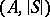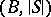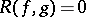# Elimination of quantifiers

(diff) ← Older revision | Latest revision (diff) | Newer revision → (diff)
An elementary theoryis said to admit elimination of quantifiers if every formula inis equivalent in the language ofto a formula without quantifiers (having the same free variables). This holds if and only ifis substructure complete, i.e., for every two modelsandofwith a common substructure, the expansionsandare elementarily equivalent in the first-order language of signature, wheredenotes the signature of(cf. Model theory; Structure). Ifis substructure complete, then it is model complete. To see this, setfor every modelofwhich is a substructure of a modelof.
For certain elementary theories of fields, the fact that they admit elimination of quantifiers reflects an algebraic elimination theory. Examples are the theory of algebraically closed fields (where vanishing of the resultant,, gives a quantifier-free criterion for the existence of a common zero of two polynomials,), and the theory of real closed fields (where the Sturm theorem gives a quantifier-free criterion for a polynomial to admit a root in a given interval, cf. also Real closed field).
The fact that the theory of real closed fields admits elimination of quantifiers is commonly known as the Tarski–Seidenberg theorem (see also Semi-algebraic set). It implies that the semi-algebraic sets over a real closed fieldare precisely the definable sets (with parameters from) in the first-order language of ordered fields. For the history and applications of the Tarski–Seidenberg theorem, and a remarkable discussion of the general technique of elimination of quantifiers, see [a2] and the literature cited therein. For applications of elimination of quantifiers to real and-adic semi- and subanalytic sets, see [a1].